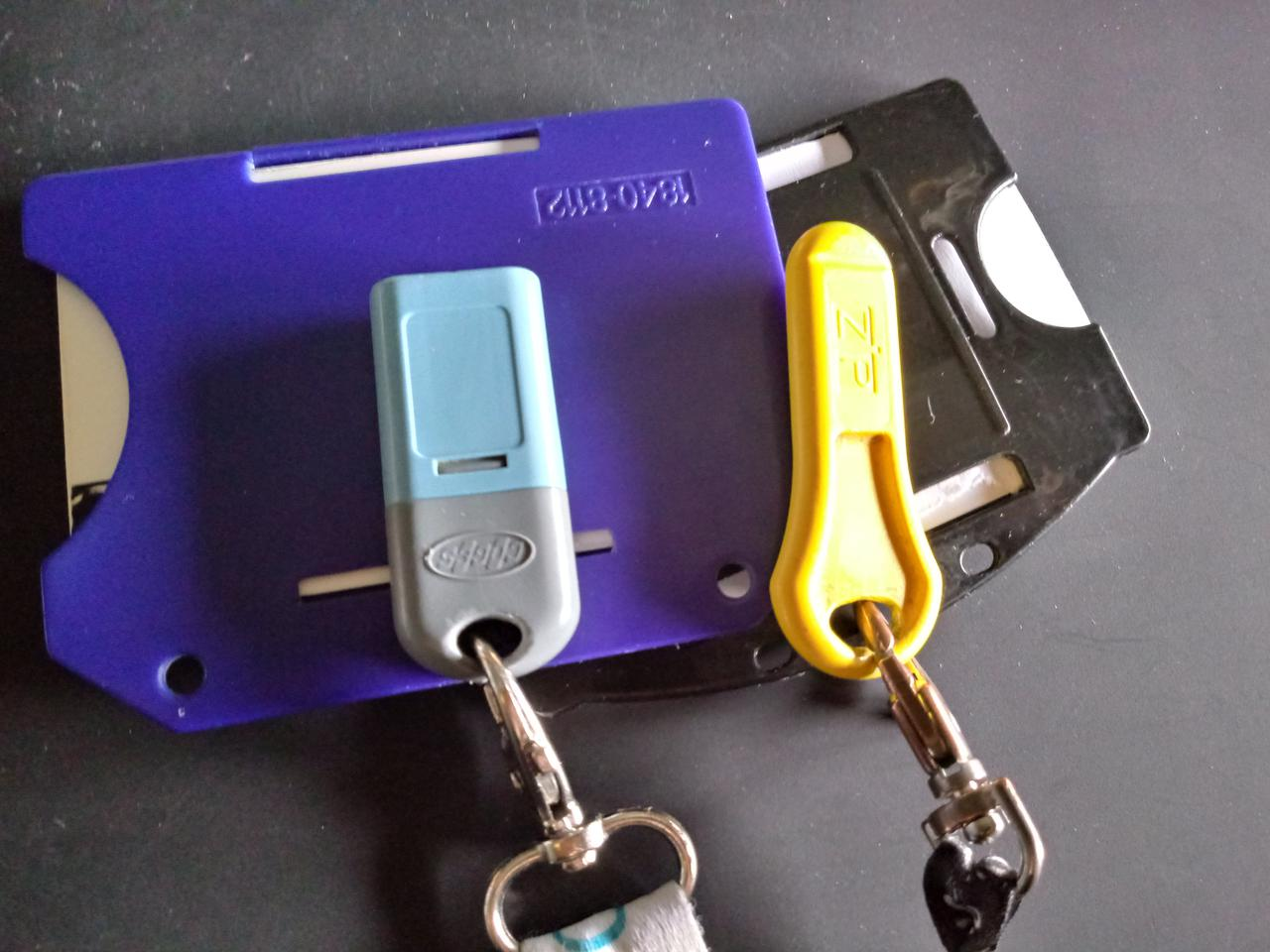# Emptying Cashless Keys with MinizincSince I bought my first cashless key to buy coffee at the company's vending machine, I never managed to spend every cent in the key - always fell short of 3 or 5 cents. I always wondered: which/how many drinks should I buy to reach zero? We will use Minizinc to answer this question.

## What is Minizinc?

Minizinc is a free and open source constraint modeling language. It can be used to formally describe (model) constraint satisfaction and optimization problems in a high-level, solver-independent way. It is available for all major platforms as a command line tool, or bundled with an IDE, and several solvers.

In our case, we're going to solve an optimization problem! An optimization problem is the problem of finding the best solution from all solutions that respect the problem's constraints (feasible solutions). How do we define the best solution, though? We use an objective function: a function that, given a feasible solution, returns its value in the system we're studying.

Don't worry if you don't understand every single word right now, I'm going to explain them in a bit!

## Let's write the model!

So, let's start by defining the parameters of our problem. We have a given amount in our key, and a list of beverages and their cost.

```% the initial amount in our cashless key
int: START_AMOUNT;
% the list of beverages (coffee, tea, chocolate, ...)
enum BEVERAGES;
% how much does a beverage cost?
array[BEVERAGES] of int: COST;
```

Let's then define how we should spend our money! Our solution is defined as an array of integers - for each beverage b, quantity[b] is the number of times I have to drink b.

```/* how many times do I have to drink a beverage B? */
array[BEVERAGES] of var int: quantity;
```

After defining the structure of the solution, we need to limit the values we can assign to the solution. Assignments to quantity must follow some rules - in this case, we must drink all beverages at least zero times, and we must spend every single cent we have. Every assignment that follows those rules is a feasible solution for the problem.

```/* constraint: cannot drink a negative amount of drinks! */
constraint forall(b in BEVERAGES)(quantity[b] >= 0);

/* constraint: we must spend exactly START_AMOUNT */
var int: spent = sum(b in BEVERAGES)(quantity[b] * COST[b]);
constraint START_AMOUNT - spent = 0;
```

At the end, we want to check how we are going to spend our money. Our objective function is how many drinks we are going to buy. We want to find an assignment of quantity that maximizes the number of coffees we have to drink - hard earned money must not be wasted!

```/* the objective function! */
var int: how_many_drinks;
constraint how_many_drinks = sum(b in BEVERAGES)(quantity[b]);

% use `maximize` to drink as many coffees as possible
% use `minimize` to cut down on coffees and get to zero euro as fast as possible
solve maximize how_many_drinks;
```

## Results

Let's feed our model with a simplified instance of the problem: it will tell us that we can buy 8 coffees and 2 chocolates, for a total of 10 drinks. If we want to cut down on coffees, we can order 3 tea cups and 4 ginseng coffees.

```shell % cat data.dzn
START_AMOUNT = 260; % 2.60 euro

BEVERAGES = { coffee, tea, ginseng, chocolate };
COST      = [     25,  40,      35,        30 ];
shell % minizinc model.mzn --data data.dzn
quantity = array1d(BEVERAGES, [8, 0, 0, 2]);
how_many_drinks = 10;
----------
==========
shell % # update the model to solve the minimization problem
shell % minizinc model.mzn --data data.dzn --all
quantity = array1d(BEVERAGES, [8, 0, 0, 2]);
how_many_drinks = 10;
----------
quantity = array1d(BEVERAGES, [2, 0, 0, 7]);
how_many_drinks = 9;
----------
quantity = array1d(BEVERAGES, [0, 0, 4, 4]);
how_many_drinks = 8;
----------
quantity = array1d(BEVERAGES, [0, 3, 4, 0]);
how_many_drinks = 7;
----------
==========
```

Nothing stops us from adding more constraints, such as "I need to drink at least one chocolate", or "I don't want to buy more than one cup of tea", or that the number of drinks must be even because I offer coffees to a colleague. Try to experiment, add your own constraints, add more beverages and modify the prices!